# In a Flash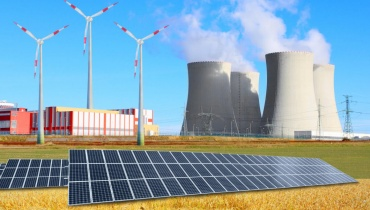Making smarter lighting choices can save the amount of energy used.

## Energy explained

Energy is literally everywhere! It's all around us and even inside of us. Our whole universe is made from energy.

There are many different types of energy – from light and heat energy to gravity, chemical energy, and electrical energy. We all use a combination of different types of energy every day.

Energy does all sorts of things for us. It moves our cars, cooks our food, plays our favourite songs, and provides light and heat for our homes. Energy also helps our bodies grow and our minds think.

So energy is a changing, doing, moving, working thing!

# Forms of Energy

Energy can be divided into two main forms: Kinetic Energy and Potential Energy.### Kinetic Energy

Kinetic energy is the energy in moving objects, also known as mass. Take wind energy – the gas molecules in the air are moving, which gives them kinetic energy.### Potential Energy

Potential energy is any form of energy that can be stored for future use. For example, water stored in a dam to be used to generate hydroelectricity is a form of potential energy.

# Types of Energy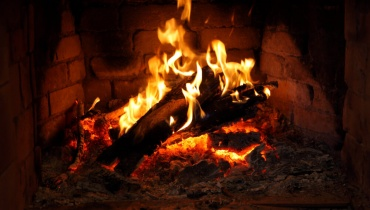### Thermal (Heat) Energy

Thermal energy is created from the vibration of atoms and molecules within substances. The faster they move, the more energy they possess and the hotter they become. Thermal energy is also called heat energy.

Let's go! >### Chemical Energy

Chemical energy is stored in the bonds of atoms and molecules – it is the energy that holds these particles together. Stored chemical energy is found in food, biomass, petroleum, and natural gas.

Let's go! >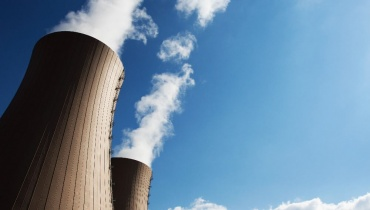### Nuclear Energy

Nuclear energy is stored in the nucleus of atoms. This energy is released when the nuclei are combined (fusion) or split apart (fission). Nuclear power plants split the nuclei of uranium atoms to produce electricity.

Let's go! >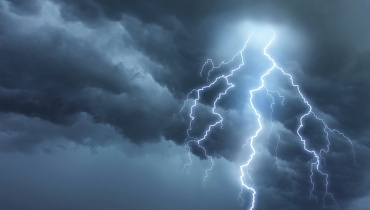### Electrical Energy

Electrical energy is the movement of electrons (the tiny particles that makeup atoms, along with protons and neutrons). Electrons that move through a wire are called electricity. Lightning is another example of electrical energy.

Let's go! >Also known as light energy or electromagnetic energy, radiant energy is a type of kinetic energy that travels in waves. Examples include the energy from the sun, x-rays, and radio waves.

Let's go! >### Light Energy

Light energy is a form of electromagnetic radiation. Light consists of photons, which are produced when an object's atoms heat up. Light travels in waves and is the only form of energy visible to the human eye.

Let's go! >### Motion Energy

Motion energy – or mechanical energy – is the energy stored in objects; as objects move faster, more energy is stored. Examples of motion energy include wind, a flowing river, a moving car, or a person running.

Let's go! >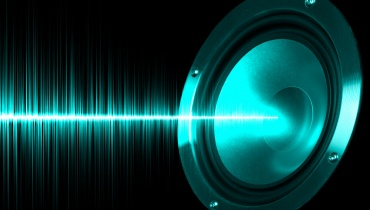### Sound Energy

Sound energy is the movement of energy through substances. It moves in waves and is produced when a force makes an object or substance vibrate. There is usually much less energy in sound than in other forms of energy.

Let's go! >### Elastic Energy

Elastic energy is a form of potential energy that is stored in an elastic object - such as a coiled spring or a stretched elastic band. Elastic objects store elastic energy when a force causes them to be stretched or squashed.

Let's go! >### Gravitational Energy

Gravitational energy is a form of potential energy. It is an energy associated with gravity or gravitational force – in other words, the energy held by an object when it is in a high position compared to a lower position.

Let's go! >

# What Do You Mean?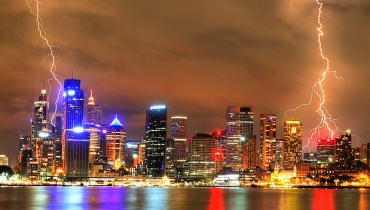Energy is never created and never destroyed.

Energy can be transferred from one object to another.

Energy comes in many different forms, which can generally be divided into two main types: potential energy and kinetic energy.

Each type of energy can be converted into any other type of energy.

Energy is never created and never destroyed – this is called the First Law of Thermodynamics.

# Energy Efficiency

Energy efficiency is the amount of useful energy you can get out of a system.

If there was a machine that was 100% energy-efficient, this means it would change all of the energy you put into it into useful energy (energy we use for a purpose).

However, when we convert one form of energy into another form of energy, it always involves a loss of usable energy, usually in the form of heat (thermal) energy.

Get the lowdown on Energy Efficiency.

# Teacher's Toolkit

Take this to the classroom!